## The idea

Problems that exhibit high initial station variation produce high variance policy-gradient estimates and are hard to solve via direct policy or value function optimization. This paper provides a novel algorithm that partitions the initial state into “slices” and optimizes an ensemble of policies over these slices, which are then unified into a central policy.

## The method

Initial states are sampled and then clustered using k-means into contexts $$\omega_i$$, each associated with a policy $$\pi_i(s,a) = \pi((\omega_i,s),a)$$. A central policy is defined as $$\pi_c(s,a)=\sum _{\omega\in\Omega} p({\omega}{\mid}s) \pi_{\omega} (s,a)$$ Each policy should stay as close to the central policy as possible by maximizing $$\eta(\pi_i) - \alpha \mathbb{E}[D_{KL}(\pi_i{\mid}{\mid}\pi_c)] {\forall i}$$. They also want to keep the divergence between policies w.r.t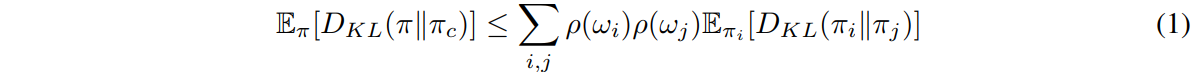To update the policies, the authors use the following loss, devired from TRPO1: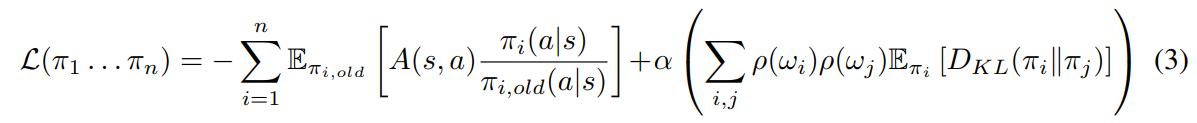Then, the central policy is updated (the authors call this the “distillation step”):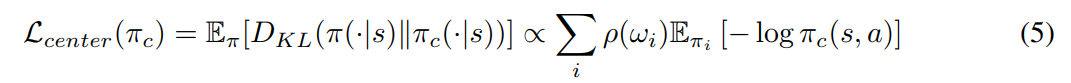Finally, here is the full algorithm: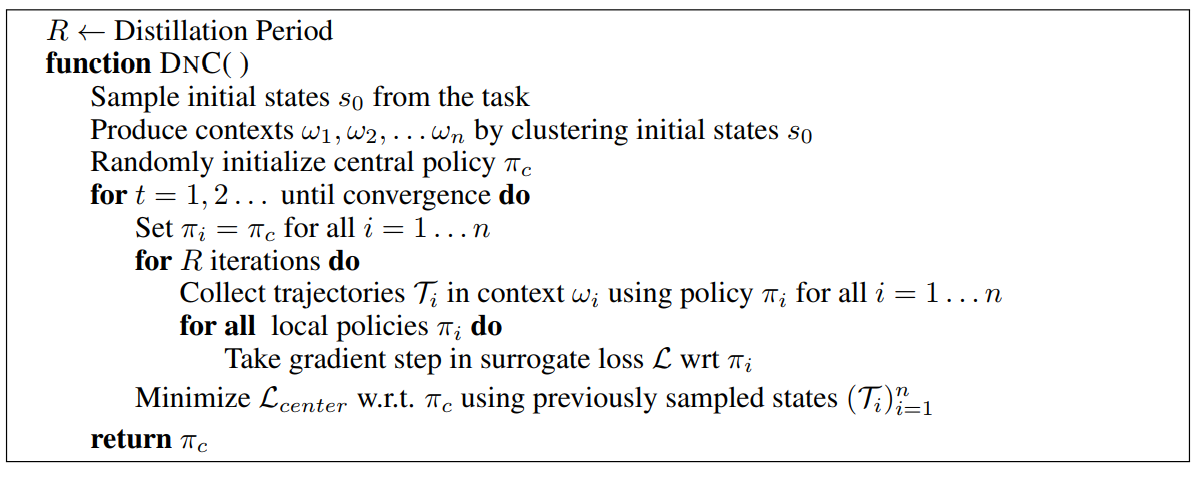## The results

• TRPO is considered state of the art in reinforcement learning Distral is another RL algorithm that splits the context, but uses a central policy learned through supervised learning
• Unconstrained DnC means that DnC is executed without KL constraints. This reduces to running TRPO on local policies.
• Centralized DnC is like running Distral but still updating the central algorithm.
• Picking requires the robotic arm to pick up a randomly-placed block and lift it as high as possible
• Lobbing requires picking a block and “lobbing” it in a randomly placed square
• Catching requires the arm to catch a ball that is thrown at it at a random velocity and initial position
• Ant requires controlling an ant to walk to a random flagged position
• Stairs requires a bipedal robot to climb a set of stairs of varying heights and lengths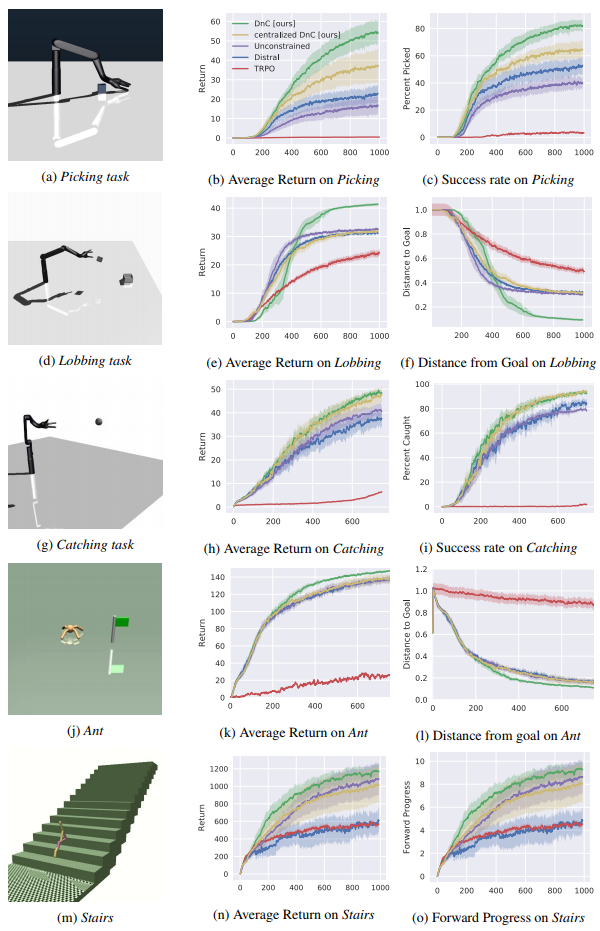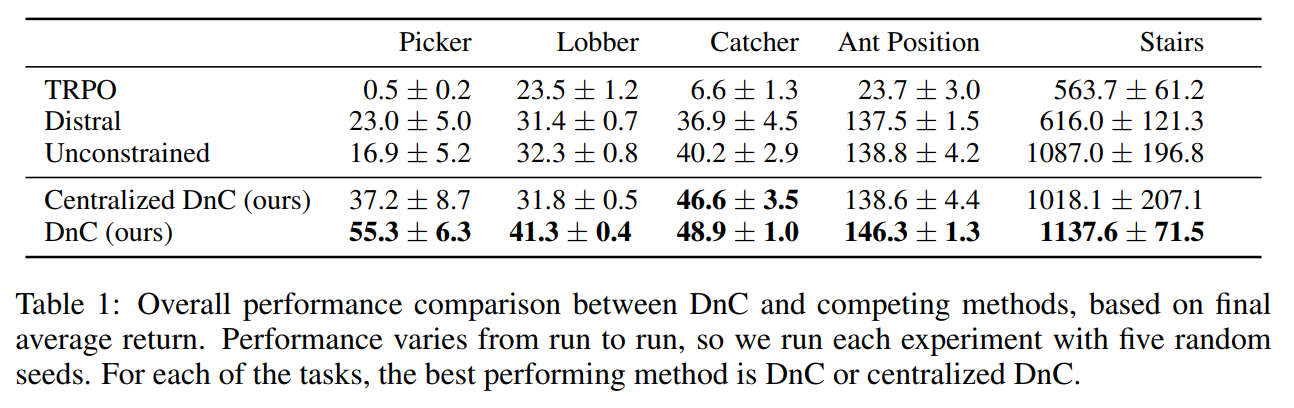Finally, here’s a video of DnC versus TRPO